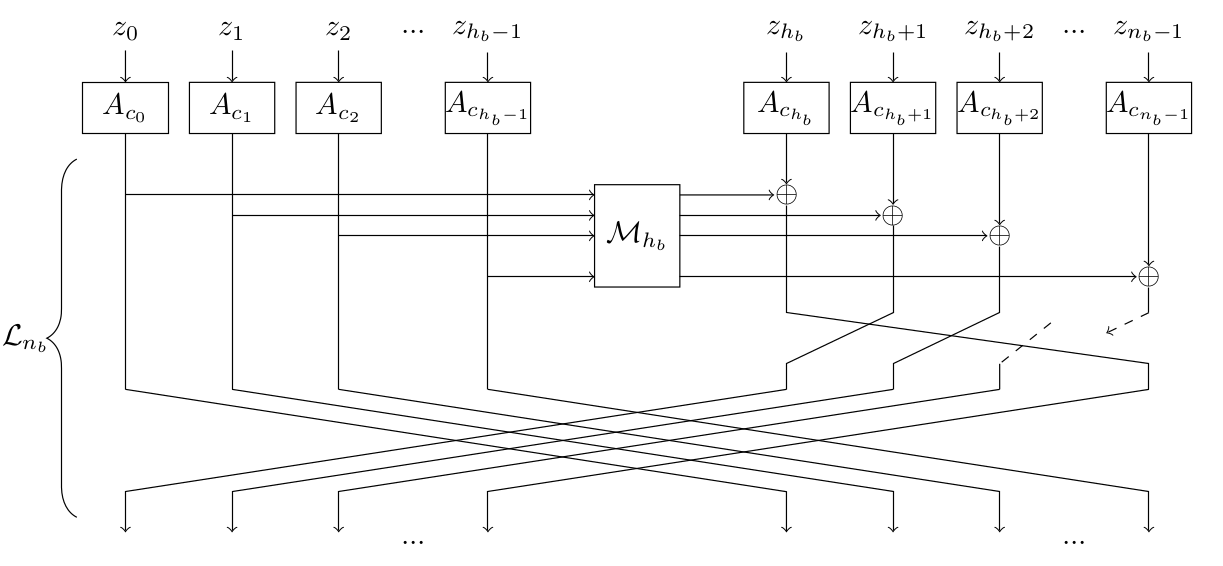# Sparkle, a lightweight cryptographic permutation

Sparkle is a family of cryptographic permutations, each operating on a different block size (256, 384 or 512 bits). They rely only on Addition, Rotations and XORs (ARX paradigm). It is possible to write a unique implementation for all variants that simply takes the block size and the number of steps as inputs. It relies on the 64-bit transformation called Alzette. We denote it $$A_c$$. The following constants are used to instantiate Alzette in Sparkle:

$c_0 = \mathtt{b7e15162}, c_1 = \mathtt{bf715880}, c_2 = \mathtt{38b4da56}, c_3 = \mathtt{324e7738},$ $c_4 = \mathtt{bb1185eb}, c_5 = \mathtt{4f7c7b57}, c_6 = \mathtt{cfbfa1c8}, c_7 = \mathtt{c2b3293d}.$

The picture below represents the round function of a Sparkle instance operating on $$64×n_b$$ bits (note $$h_n = n_n / 2$$).The following code is a universal reference implementation of the Sparkle permutation in the C language. It accepts the input state, the total number $$n_b$$ of branches, and the number $$n_s$$ of steps. In particular, the following parameters are recommended:

• for Sparkle256: $$n_b=4$$, $$n_s = 10$$ (full version) and $$n_s = 7$$ (slim version);
• for Sparkle384: $$n_b=6$$, $$n_s = 11$$ (full version) and $$n_s = 7$$ (slim version);
• for Sparkle512: $$n_b=8$$, $$n_s = 12$$ (full version) and $$n_s = 8$$ (slim version).
#define MAX_BRANCHES 8
#define ROT(x, n) (((x) >> (n)) | ((x) << (32-(n))))
#define ELL(x) (ROT(((x) ^ ((x) << 16)), 16))
#define ALZETTE_ROUND(x, y, s, t, c) \
(x) += ROT((y), (s));            \
(y) ^= ROT((x), (t));            \
(x) ^= (c);

// Round constants
static const uint32_t RCON[MAX_BRANCHES] = {      \
0xB7E15162, 0xBF715880, 0x38B4DA56, 0x324E7738, \
0xBB1185EB, 0x4F7C7B57, 0xCFBFA1C8, 0xC2B3293D  \
};

void sparkle(uint32_t *state, int nb, int ns)
{
int i, j;  // Step and branch counter
uint32_t rc, tmpx, tmpy, x0, y0;

for(i = 0; i < ns; i ++) {
state ^= RCON[i%MAX_BRANCHES]; state ^= i;
// ARXBox layer
for(j = 0; j < 2*nb; j += 2) {
rc = RCON[j>>1];
ALZETTE_ROUND(state[j], state[j+1], 31, 24, rc);
ALZETTE_ROUND(state[j], state[j+1], 17, 17, rc);
ALZETTE_ROUND(state[j], state[j+1],  0, 31, rc);
ALZETTE_ROUND(state[j], state[j+1], 24, 16, rc);
}
// Linear layer
tmpx = x0 = state;
tmpy = y0 = state;
for(j = 2; j < nb; j += 2) {
tmpx ^= state[j]; tmpy ^= state[j+1];
}
tmpx = ELL(tmpx); tmpy = ELL(tmpy);
for (j = 2; j < nb; j += 2) {
state[j-2] = state[j+nb] ^ state[j] ^ tmpy;
state[j+nb] = state[j];
state[j-1] = state[j+nb+1] ^ state[j+1] ^ tmpx;
state[j+nb+1] = state[j+1];
}
state[nb-2] = state[nb] ^ x0 ^ tmpy; state[nb] = x0;
state[nb-1] = state[nb+1] ^ y0 ^ tmpx; state[nb+1] = y0;
}
}